## Topic: Fractions

Free Printable Worksheets Using Fractions

## Fraction Addition with Same Denominators (Set 1)Math worksheet – fractions with the same denominators. Write the fractions below the pictures and add them. Color the sum in the picture and write the correct fraction under it....

## Fraction Addition with Same Denominators (Set 2)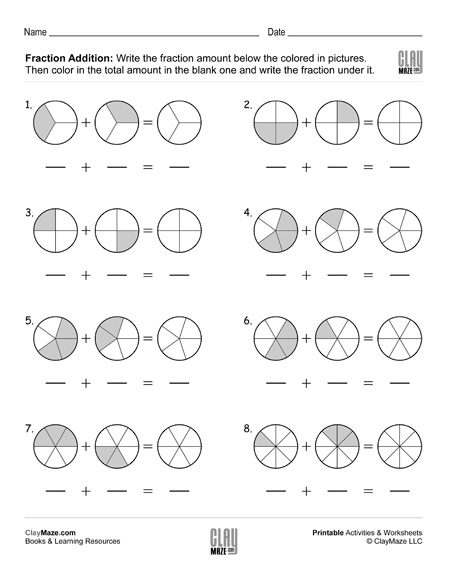Fractions worksheet with same denominators. Write the fraction amounts under the pictures. Color the total fraction amount in the blank picture and write the sum under it....

## Fraction Addition with Same Denominators (Set 3)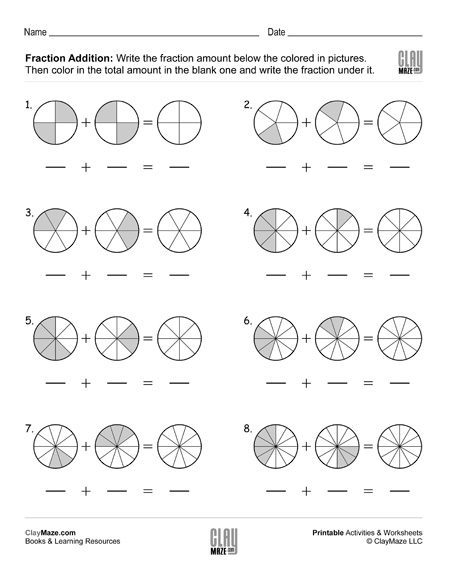Adding fractions with common denominator worksheet. Add the fractions and color the sum in the picture....

## Fraction Addition with Same Denominators (Set 4)Fraction worksheet – Write the fraction amount under he picture. Then color in the total fraction amount and write the sum in the blank below it....

## Fraction Addition with Same Denominators (Set 5)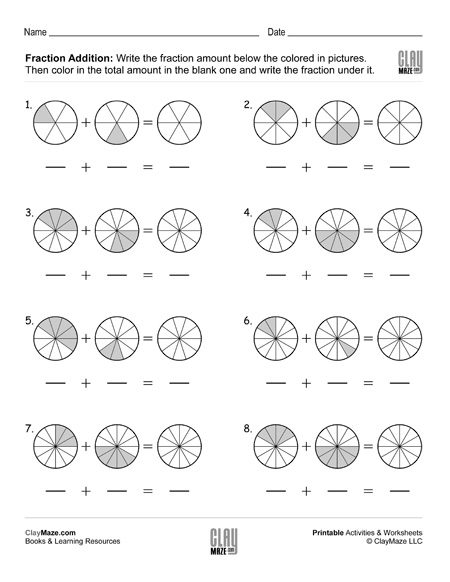Fraction math: addition – Write the fraction amounts below the pictures. Color in the total amount in the blank and write the amount below it.  ...

## Fraction Subtraction with Same Denominators (Set 1)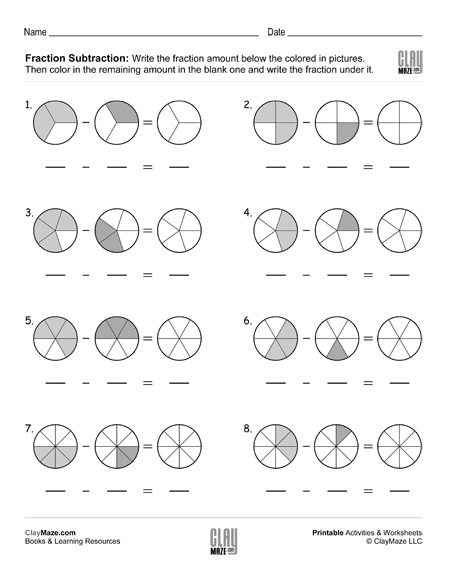Subtracting fractions worksheet – Write the fraction amount below the picture. Subtract the fractions and color in the remaining amount in the picture....

## Fraction Subtraction with Same Denominators (Set 2)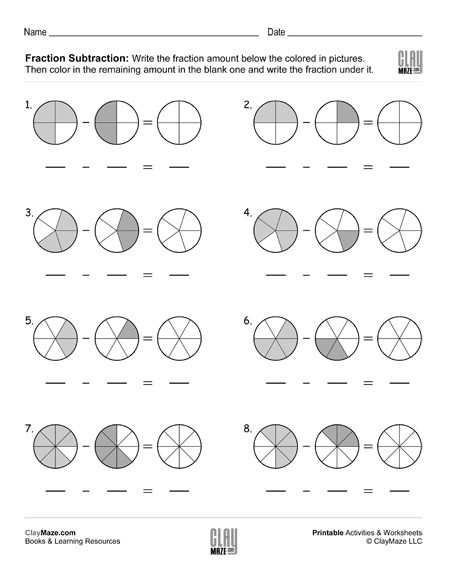Fraction subtraction with common denominators – write the fractions, then subtract them using the pictures and color in the correct amount....

## Fraction Subtraction with Same Denominators (Set 3)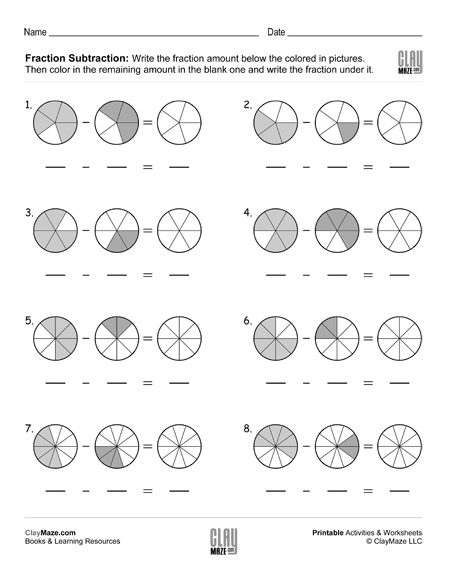Subtracting fractions free, printable worksheet – Write the fractions, subtract them and color the remaining amount....

## Fraction Subtraction with Same Denominators (Set 4)Fraction subtraction with common denominators worksheet – Write the correct fraction amount under each picture. Subtract the fractions and color the fractional amount in the blank picture....

## Color the Fractions (Set 1)Color in the correct fraction amount in the pictures. Recommended for kindergarten and first grade....

## Color the Fractions (Set 2)Download this free, printable worksheet for fraction practice. Color the fraction amounts....

## Color the Fractions (Set 3)Fraction practice worksheet. Color the correct fraction amounts. Recommended for first grade and second grade. (Note: The number of slices is higher than the denominator.)...

## Fraction Addition and Simplifying with Coloring (Set 1)Add the fractions and color the correct amount. Simplify the sum....

## Fraction Addition and Simplifying with Coloring (Set 2)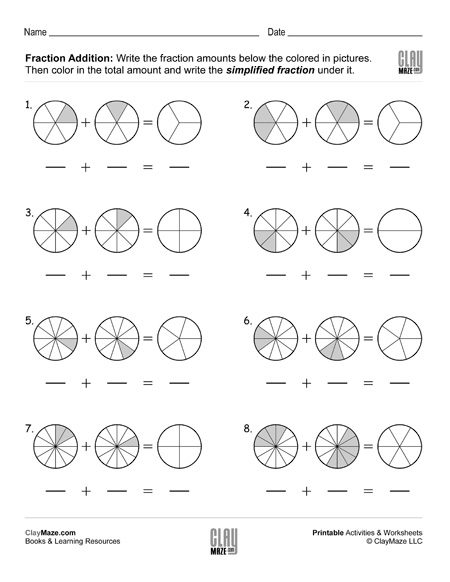Add the fractions and color the correct amount. Simplify the sum....

## Simplify the Fractions and Color the Amount (Set 1)This worksheet is for children learning how to simplify fractions. It includes pictures to color in to help see how they are simplified  visually. Simplify the fractions and color the...